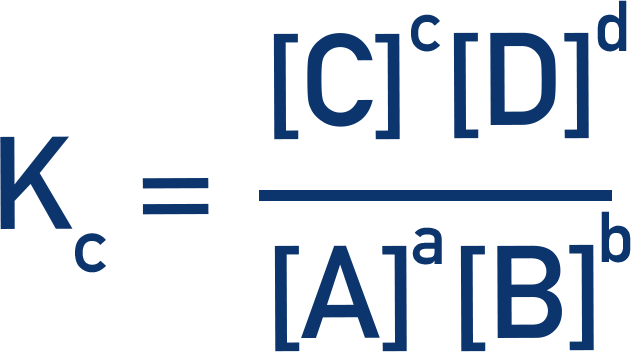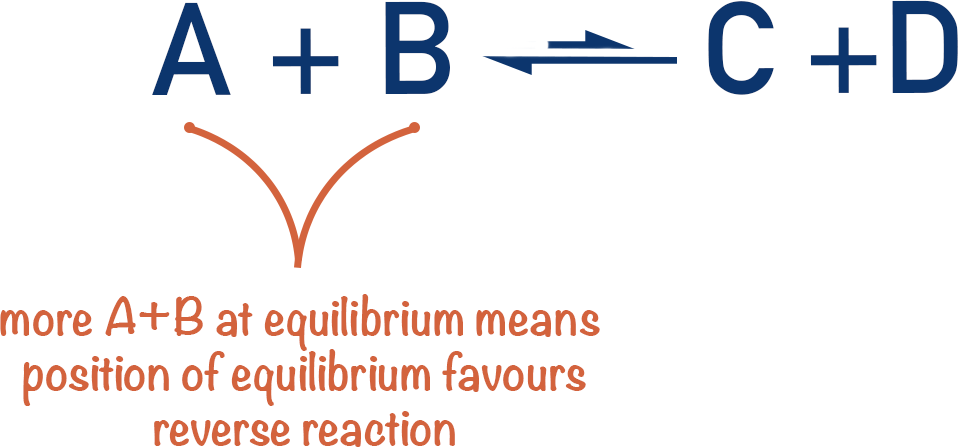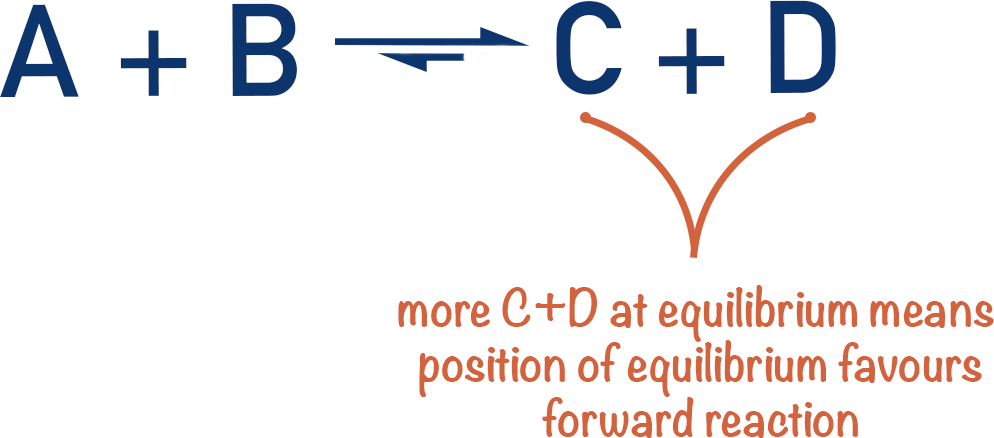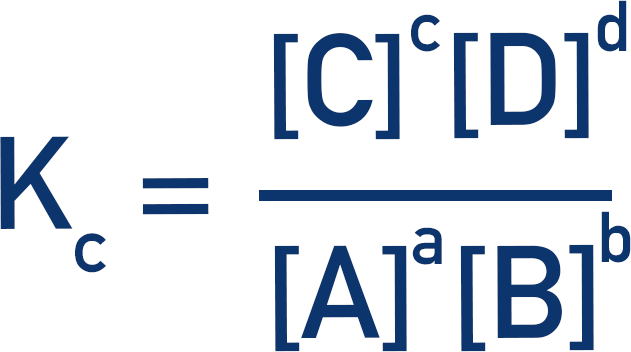Quick Notes Equilibrium Constant

• Equilibrium of a reversible reaction is achieved when the concentrations of both the reactants and products remain constant.
• At equilibrium, the rate of the forward reaction is the same as the rate of the backward reaction.
• The ‘position of equilibrium’ describes how much either the forward or reverse reaction was favoured before equilibrium was reached.
• This can be shown mathematically by comparing the concentrations of reactants to products at equilibrium.
• The equilibrium constant, Kc, is a constant that describes the ratio between reactants and products at equilibrium.
• It is calculated by dividing the concentrations of the products by the concentrations of the reactants (raised to the power of their molar ratios).• Kc values are only for an equilibrium at a specific temperature, if the temperature of an equilibrium system is changed, the value of Kc will also change.

Full Notes Equilibrium Constant

When the concentrations of reactants and products reacting in a reversible reaction no longer change, equilibrium is reached. The ‘position of equilibrium’ describes how much either the forward or reverse reaction was favoured before equilibrium was reached. Favoured means which reaction happens more.

Equilibrium constants (Kc) can be used to show this position.

Imagine a simple equilibrium between A + B and C + D.

If you have more A + B at equilibrium, the backward reaction must have been favoured more than the forward reaction before equilibrium was reached. We would describe this by saying the position of equilibrium lies to the left (backward reaction favoured).If you have more C + D at equilibrium, the ‘position of equilibrium’ must lie to the right (forward reaction favoured).From this, we can see that by comparing the amount of A + B to C + D (at equilibrium), we can determine whether the position of equilibrium lies to the left or right. Mathematically, we use a value to show this. This value is called an equilibrium constant, shown as ‘Kc’.

The equilibrium constant, Kc, is a constant that describes the ratio between reactants and products at equilibrium.

Kc values are calculated by dividing the concentrations of products by the concentrations of reactants (raised to the power of their molar ratios).Where a, b, c and d are the molar ratios of each reactant in the reaction.

If the amounts of A + B and C + D are the same, the top of the fraction will be the same as the bottom, so Kc will have a value of exactly one. This would show a position of equilibrium that is perfectly in the middle, favouring the forward and backward reactions equally.

If there is more C + D at equilibrium than A + B, the top of the fraction will be larger than the bottom, so Kc will have a value greater than one. This would show a position of equilibrium that lies to the right (is in favour of the forward reaction). The larger the value of Kc (over one), the more the position of equilibrium favours the forward reaction.

If there is more A + B at equilibrium than C + D, the bottom of the fraction will be larger than the top, so Kc will have a value smaller than one. This would show a position of equilibrium that lies to the left (is in favour of the reverse reaction). The smaller the value of Kc (under one), the more the position of equilibrium favours the reverse reaction.

It’s important to understand that Kc doesn’t give any information about how fast the reactions happen; it just gives information about the balance between the forward and reverse reactions before equilibrium is reached.

Changing the concentrations of the reactants and products doesn’t change the value of Kc for a reaction. Only temperature changes the value of Kc. The units for Kc can also be different for different equilibrium systems.